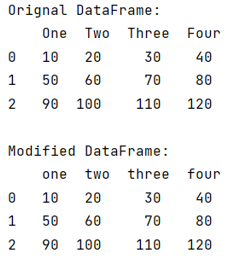# How to make pandas DataFrame column headers all lowercase?

Given a Pandas DataFrame, we have to make its column headers all lowercase. By Pranit Sharma Last updated : September 19, 2023

Pandas is a special tool that allows us to perform complex manipulations of data effectively and efficiently. Inside pandas, we mostly deal with a dataset in the form of DataFrame. DataFrames are 2-dimensional data structures in pandas. DataFrames consists of rows, columns, and the data.

Columns are the different fields that contain their particular values when we create a DataFrame. We can perform certain operations on both rows & column values. Each column has a specific header/name.

## Problem statement

Given a Pandas DataFrame, we have to make its column headers all lowercase.

## Making pandas DataFrame column headers all lowercase

To convert all the column headers into lower case, we will use str.lower() method where str is nothing but each of the column header.

Note

To work with pandas, we need to import pandas package first, below is the syntax:

```import pandas as pd
```

Let us understand with the help of an example,

## Python program to make pandas DataFrame column headers all lowercase

```# Importing pandas package
import pandas as pd

# Importing numpy package
import numpy as np

# Create a DataFrame
df = pd.DataFrame([
[10,20,30,40],
[50,60,70,80],
[90,100,110,120]
],
columns=['One','Two','Three','Four']
)

# Display original DataFrame
print("Orignal DataFrame:\n",df,"\n")

# Converting all the header name into lower case
df.columns = [x.lower() for x in df.columns]

# Display modified DataFrame
print("Modified DataFrame:\n",df)
```

### Output

The output of the above program is: